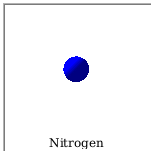Stick Triangle - Maple Help

Stick Triangle

Main Concept

Suppose you break a stick into three pieces and try to assemble them into a triangle. If you choose the two breaking points at random points in the interval, the theoretical probability that the pieces are able to form a triangle is $\frac{1}{4}$ or 25%.  To see how well this theoretical probability works in practice, select two points on the line below at random by clicking on the image then click 'Assemble Triangle' to see if a triangle can be made out of those pieces. To try to make another triangle yourself, click 'Start a new triangle' and select new breaking points. You can also let Maple generate random sets of breaking points.Explanation of probability Assume the endpoints of the stick are at the points 0 and 1. Let $x$ and $y$ be the two breaking points, chosen at random from the values in the interval . Let us assume at first that . The three sides of the triangle have lengths $x$, $y-x$, and $1-y$. The three conditions that must be satisfied for a triangle to be formed are thus:   , , . Simplifying these inequalities gives us: , , and . We can rearrange this to give: , . The total probability for this case can thus be given by the integral: . Considering that the other case (that ) is equally likely, the total probability that a triangle can be formed is .n =More MathApps